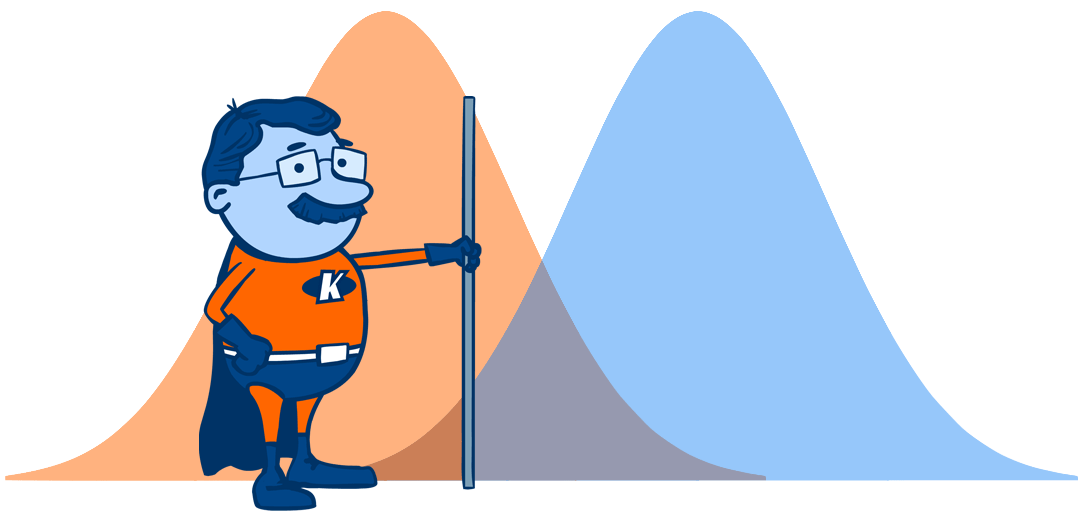Simple StatisticsIt is easy to make appropriate statistical decisions for small datasets without being immersed in complex data analysis.

The main statistics are easy to do in Excel or SPSS:

• Calculating means and standard deviations
• Carrying out t-tests and analysis of variance to compare means between sets of data
• Calculating correlation coefficients to enable you to see the relationship between two sets of scores
• Using contingency tables to determine how different groups can be categorised.

Statistical decisions are just really about making a judgement and then expressing how confident you are in this judgement. Statistical analysis provides a method of calculating confidence in decisions you reach, rather than just asking a reader to trust you.

The first thing you need to decide is what sort of statistical decision you want to make. You might want to see how variables relate to each other, such as whether shoe size relates to height. Or you might want to judge whether numbers in one set of data are bigger or smaller than numbers in another set of data. Here is a short video from the folks at The Statistics Learning Centre that explains these things really simply:

If you are familiar with basic statistical concepts then you can use simple decision trees such as the one illustrated below to help identify the type of test that you need to perform. This chart applies to parametric data, which was explained in the short video above.

Once you've identified the required statistical test, there are a lot of widely available resources that provide step-by-step instructions on how to run these tests using different software. For example, Andy Field's SPSS Tutorials and the The RMUoHP Biostatistics Resource Channel do a great job of explaining how to conduct some of these tests in SPSS.

If you're searching for a particular statistical test on YouTube, just make sure you type in as precise a search terms as you can. For example:

• t-test for independent samples
• t-test using SPSS
• correlation coefficients in EXCEL

Try to give the test by name and, if appropriate, the software on which you want to conduct the calculations.

Pin It on Pinterest